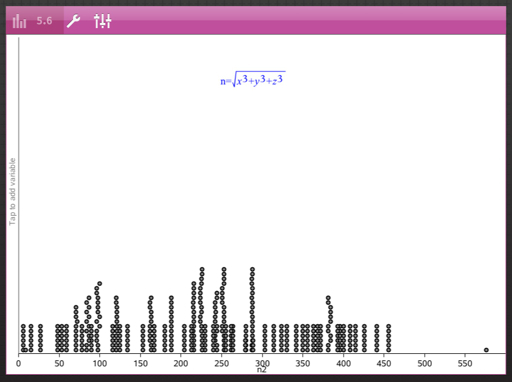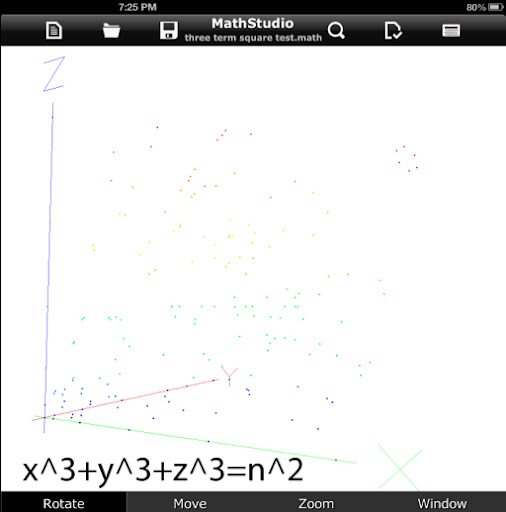## Wednesday, July 10, 2013

### x^3+ y^3 + z^3 = n^2

The goal is to find solutions of x^3+ y^3 + z^3 = n^2 where x, y, z, and n are integers greater than 1.

Using the following program to find solutions (TI nSpire App):

Define test32(k,n)=
Prgm
:x2:={}
:y2:={}
:z2:={}
:n2:={}
:For a,k,n
:For b,k,n
:For c,k,n
:d:=(a^(3)+b^(3)+c^(3))^(1/2)
:If fPart(d)=0 Then
:x2:=augment(x2,{a})
:y2:=augment(y2,{b})
:z2:=augment(z2,{c})
:n2:=augment(n2,{d})
:EndIf
:EndFor
:EndFor
:EndFor
:EndPrgm

Some solutions for testing integers from 1 to 50:
1^3 + 2^3 + 6^3 = 225 = 15^2
1^3 + 4^3 + 14^3 = 2809 = 53^2
2^3 + 12^3 + 50^3 = 126,736 = 356^2
3^3 + 3^3 + 3^3 = 81 = 9^2
4^3 + 4^3 + 17^3 = 5041 = 71^2
4^3 + 17^3 + 22^3 = 15,625 = 125^2
8^3 + 4^3 + 12^3 = 2304 = 48^2
8^3 + 13^3 + 15^3 = 6084 = 78^2
8^3 + 30^3 + 49^3 = 145,161 = 381^2
10^3 + 10^3 + 20^3 = 10,000 = 100^2
10^3 + 24^3 + 26^3 = 32,400 = 180^2
12^3 + 12^3 + 12^3 = 5184 = 72^2

In the range of 1 ≤ x ≤ 25, 1 ≤ y ≤ 25, and 1 ≤ z ≤ 25, there are 122 solutions.

In the range of 1 ≤ x ≤ 50, 1 ≤ y ≤ 50, and 1 ≤ z ≤ 50, there are 415 solutions. Presented next is a graph of √(x^3 + y^3 + z^3) and where square root of the sums stack up:Finally, using the MathStudio app, is a plot of solutions for 0 ≤ x ≤ 25, 0 ≤ y ≤ 25, and 0 ≤ z ≤ 25.Until next time,

Eddie

### Casio fx-CG50: Sparse Matrix Builder

Casio fx-CG50: Sparse Matrix Builder Introduction The programs can create a sparse matrix, a matrix where most of the entries have zero valu...